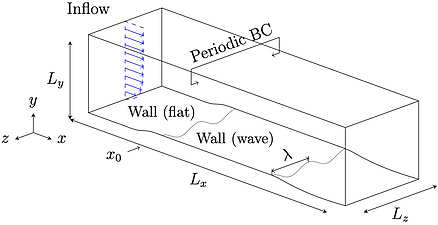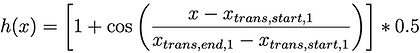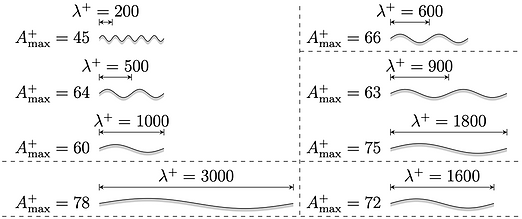# Actuated turbulent boundary layer flows

This data collection contains time-series of three-dimensional snapshots for a parametric study of flat plate turbulent boundary layer flow actuated by spanwise traveling transversal surface waves with the goal of friction drag reduction. In the following some details regarding the numerical setup and the structure of the data is given.

• A full description of the numerical setup and the parametric study is presented in .

• The non-actuated turbulent boundary layer flow has a momentum thickness Reynolds number of around Reθ=1000.

• The numerical method discretizes the compressible Navier-Stokes equations at a Mach number of M = 0.1, i.e., the flows can be regarded practically incompressible.

• A total of 79 variations of the actuation parameters wavelength, period, and amplitude were considered. To save computational effort shorter wavelengths were partially simulated in domains which are narrower than the maximum wavelength. That is, six different domain widths were considered, therefore there are six different grids, one for each domain width.# Simulation setup# Figure 2: Overview of the computational domain of the actuated flat plate. The incoming flow (blue) is created by an synthetic turbulence generation method (STG) .

Wall actuation function:

Transition function at the start (flat wall to actuated wall):

Transition function at the end (actuated wall to flat wall):# Figure 3: Sideview of the actuated wall showing the two transition zones at the start and at the end.# Description of the dataset

For every case an HDF5 file containing [u, v, w, ρ, p] is written out every ∆n = 300 time steps. The file includes only cells that are in the actuated region. Each snapshot is containted within a single boxOutputXYZ.hdf5 file, where XYZ is the time step n at which the snapshot was written out. Each boxOutput-file contains the following structure:

boxOutput250200.hdf5 :

−> /t250200

−> time             [ attribute of solver time ]

−> timeStep         [ attribute of solver time step ]

−> physicalTime     [ attribute of convection time ]

−> physicalTimeStep [ attribute of convection time step ]

−> /box0

−> u   [ structured dataset with (i,j,k)−indexing ]

−> v   [ structured dataset with (i,j,k)−indexing ]

−> w   [ structured dataset with (i,j,k)−indexing ]

−> rho [ structured dataset with (i,j,k)−indexing ]

−> p   [ structured dataset with (i,j,k)−indexing ]

−> offseti [ attribute of i-offset of subvolume ]

−> offsetj [ attribute of j-offset of subvolume ]

−> offsetk [ attribute of k-offset of subvolume ]

−> sizei   [ attribute of i-offset of subvolume ]

−> sizej   [ attribute of j-offset of subvolume ]

−> sizek   [ attribute of i-offset of subvolume ]

Works using this datset need to cite this manuscript:

  Albers, M., Meysonnat, P. S., Fernex, D., Semaan, R., Noack, B. R., & Schröder, W. (2020). Drag Reduction and Energy

Saving by Spanwise Traveling Transversal Surface Waves for Flat Plate Flow. Flow, Turbulence and Combustion, 105(1),

Further reference:

  Roidl, B., Meinke, M., & Schröder, W. (2013). A reformulated synthetic turbulence generation method for a zonal RANS–LES

method and its application to zero-pressure gradient boundary layers. International Journal of Heat and Fluid Flow, 44, 28–# Figure 5: Dimensions of the subpartition of the numerical domain (box) for which all primitive variables are written out..

computational mesh

6.3 GB

grid_TBL.tar.gz

w=1000, L=0, T=0, A=0

103 GB

TBL_w1000_L0_T0_A0.tar.gz

w=1000, L=200, T=20, A=30

65 GB

TBL_w1000_L200_T20_A30.tar.gz

w=1000, L=200, T=30, A=21

65 GB

TBL_w1000_L200_T30_A21.tar.gz

w=1000, L=200, T=40, A=30

65 GB

TBL_w1000_L200_T40_A30.tar.gz

w=1000, L=200, T=50, A=45

65 GB

TBL_w1000_L200_T50_A45.tar.gz

w=1000, L=200, T=60, A=30

65 GB

TBL_w1000_L200_T60_A30.tar.gz

w=1000, L=200, T=70, A=14

65 GB

TBL_w1000_L200_T70_A14.tar.gz

w=1000, L=200, T=70, A=38

65 GB

TBL_w1000_L200_T70_A38.tar.gz

w=1000, L=200, T=100, A=28

65 GB

TBL_w1000_L200_T100_A28.tar.gz

w=1000, L=500, T=20, A=30

65 GB

TBL_w1000_L500_T20_A30.tar.gz

w=1000, L=500, T=30, A=22

65 GB

TBL_w1000_L500_T30_A22.tar.gz

w=1000, L=500, T=40, A=21

65 GB

TBL_w1000_L500_T40_A21.tar.gz

w=1000, L=500, T=40, A=30

65 GB

TBL_w1000_L500_T40_A30.tar.gz

w=1000, L=500, T=60, A=30

65 GB

TBL_w1000_L500_T60_A30.tar.gz

w=1000, L=500, T=70, A=36

65 GB

TBL_w1000_L500_T70_A36.tar.gz

w=1000, L=500, T=70, A=64

65 GB

TBL_w1000_L500_T70_A64.tar.gz

w=1000, L=500, T=100, A=48

65 GB

TBL_w1000_L500_T100_A48.tar.gz

w=1000, L=1000, T=20, A=10

65 GB

TBL_w1000_L1000_T20_A10.tar.gz

w=1000, L=1000, T=20, A=30

65 GB

TBL_w1000_L1000_T20_A30.tar.gz

w=1000, L=1000, T=20, A=50

65 GB

TBL_w1000_L1000_T20_A50.tar.gz

w=1000, L=1000, T=40, A=10

65 GB

TBL_w1000_L1000_T40_A10.tar.gz

w=1000, L=1000, T=40, A=20

65 GB

TBL_w1000_L1000_T40_A20.tar.gz

w=1000, L=1000, T=40, A=30

65 GB

TBL_w1000_L1000_T40_A30.tar.gz

w=1000, L=1000, T=40, A=40

65 GB

TBL_w1000_L1000_T40_A40.tar.gz

w=1000, L=1000, T=40, A=50

65 GB

TBL_w1000_L1000_T40_A50.tar.gz

w=1000, L=1000, T=40, A=60

65 GB

TBL_w1000_L1000_T40_A60.tar.gz

w=1000, L=1000, T=80, A=10

65 GB

TBL_w1000_L1000_T80_A10.tar.gz

w=1000, L=1000, T=80, A=20

65 GB

TBL_w1000_L1000_T80_A20.tar.gz

w=1000, L=1000, T=80, A=30

65 GB

TBL_w1000_L1000_T80_A30.tar.gz

w=1000, L=1000, T=80, A=40

65 GB

TBL_w1000_L1000_T80_A40.tar.gz

w=1000, L=1000, T=80, A=50

65 GB

TBL_w1000_L1000_T80_A50.tar.gz

w=1000, L=1000, T=80, A=60

65 GB

TBL_w1000_L1000_T80_A60.tar.gz

w=1000, L=1000, T=120, A=10

65 GB

TBL_w1000_L1000_T120_A10.tar.gz

w=1000, L=1000, T=120, A=20

65 GB

TBL_w1000_L1000_T120_A20.tar.gz

w=1000, L=1000, T=120, A=30

65 GB

TBL_w1000_L1000_T120_A30.tar.gz

w=1000, L=1000, T=120, A=40

65 GB

TBL_w1000_L1000_T120_A40.tar.gz

w=1000, L=1000, T=120, A=50

65 GB

TBL_w1000_L1000_T120_A50.tar.gz

w=1000, L=1000, T=120, A=60

65 GB

TBL_w1000_L1000_T120_A60.tar.gz

w=1200, L=600, T=30, A=44

78 GB

TBL_w1200_L600_T30_A44.tar.gz

w=1200, L=600, T=40, A=59

78 GB

TBL_w1200_L600_T40_A59.tar.gz

w=1200, L=600, T=50, A=36

78 GB

TBL_w1200_L600_T50_A36.tar.gz

w=1200, L=600, T=60, A=21

78 GB

TBL_w1200_L600_T60_A21.tar.gz

w=1200, L=600, T=70, A=29

78 GB

TBL_w1200_L600_T70_A29.tar.gz

w=1200, L=600, T=80, A=66

78 GB

TBL_w1200_L600_T80_A66.tar.gz

w=1200, L=600, T=90, A=51

78 GB

TBL_w1200_L600_T90_A51.tar.gz

w=1200, L=600, T=100, A=14

78 GB

TBL_w1200_L600_T100_A14.tar.gz

w=1600, L=1600, T=20, A=22

103 GB

TBL_w1600_L1600_T20_A22.tar.gz

w=1600, L=1600, T=40, A=34

103 GB

TBL_w1600_L1600_T40_A34.tar.gz

w=1600, L=1600, T=40, A=48

103 GB

TBL_w1600_L1600_T40_A48.tar.gz

w=1600, L=1600, T=50, A=60

103 GB

TBL_w1600_L1600_T50_A60.tar.gz

w=1600, L=1600, T=50, A=73

103 GB

TBL_w1600_L1600_T50_A73.tar.gz

w=1600, L=1600, T=60, A=27

103 GB

TBL_w1600_L1600_T60_A27.tar.gz

w=1600, L=1600, T=70, A=71

103 GB

TBL_w1600_L1600_T70_A71.tar.gz

w=1600, L=1600, T=80, A=17

103 GB

TBL_w1600_L1600_T80_A17.tar.gz

w=1600, L=1600, T=90, A=65

103 GB

TBL_w1600_L1600_T90_A65.tar.gz

w=1600, L=1600, T=100, A=40

103 GB

TBL_w1600_L1600_T100_A40.tar.gz

w=1800, L=900, T=30, A=49

116 GB

TBL_w1800_L900_T30_A49.tar.gz

w=1800, L=900, T=40, A=63

116 GB

TBL_w1800_L900_T40_A63.tar.gz

w=1800, L=900, T=50, A=22

116 GB

TBL_w1800_L900_T50_A22.tar.gz

w=1800, L=900, T=50, A=44

116 GB

TBL_w1800_L900_T50_A44.tar.gz

w=1800, L=900, T=70, A=28

116 GB

TBL_w1800_L900_T70_A28.tar.gz

w=1800, L=900, T=80, A=17

116 GB

TBL_w1800_L900_T80_A17.tar.gz

w=1800, L=900, T=80, A=60

116 GB

TBL_w1800_L900_T80_A60.tar.gz

w=1800, L=900, T=90, A=39

116 GB

TBL_w1800_L900_T90_A39.tar.gz

w=1800, L=1800, T=30, A=14

116 GB

TBL_w1800_L1800_T30_A14.tar.gz

w=1800, L=1800, T=40, A=51

115 GB

TBL_w1800_L1800_T40_A51.tar.gz

w=1800, L=1800, T=40, A=70

115 GB

TBL_w1800_L1800_T40_A70.tar.gz

w=1800, L=1800, T=50, A=59

116 GB

TBL_w1800_L1800_T50_A59.tar.gz

w=1800, L=1800, T=60, A=44

116 GB

TBL_w1800_L1800_T60_A44.tar.gz

w=1800, L=1800, T=60, A=75

116 GB

TBL_w1800_L1800_T60_A75.tar.gz

w=1800, L=1800, T=70, A=29

116 GB

TBL_w1800_L1800_T70_A29.tar.gz

w=1800, L=1800, T=80, A=36

116 GB

TBL_w1800_L1800_T80_A36.tar.gz

w=1800, L=1800, T=90, A=66

116 GB

TBL_w1800_L1800_T90_A66.tar.gz

w=1800, L=1800, T=100, A=21

116 GB

TBL_w1800_L1800_T100_A21.tar.gz

w=3000, L=3000, T=40, A=51

192 GB

TBL_w3000_L3000_T40_A51.tar.gz

w=3000, L=3000, T=50, A=78

192 GB

TBL_w3000_L3000_T50_A78.tar.gz

w=3000, L=3000, T=60, A=26

193 GB

TBL_w3000_L3000_T60_A26.tar.gz

w=3000, L=3000, T=70, A=64

193 GB

TBL_w3000_L3000_T70_A64.tar.gz

w=3000, L=3000, T=80, A=11

194 GB

TBL_w3000_L3000_T80_A11.tar.gz